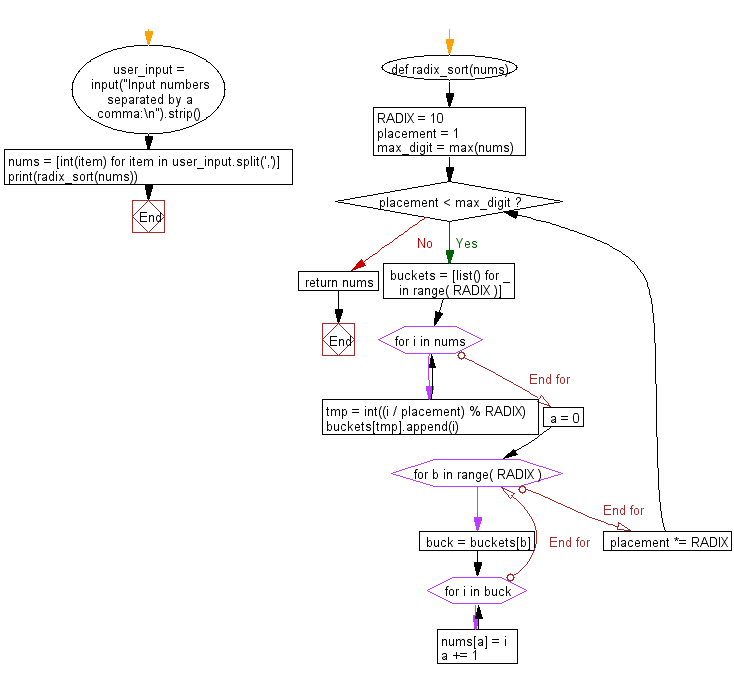﻿ Python: Sort a list of elements using Radix sort - w3resource# Python: Sort a list of elements using Radix sort

## Python Search and Sorting : Exercise-19 with Solution

Write a Python program to sort a list of elements using Radix sort.
According to Wikipedia "In computer science, radix sort is a non-comparative integer sorting algorithm that sorts data with integer keys by grouping keys by the individual digits which share the same significant position and value".

Sample Solution:

Python Code:

``````def radix_sort(nums):
placement = 1
max_digit = max(nums)

while placement < max_digit:
buckets = [list() for _ in range( RADIX )]
for i in nums:
tmp = int((i / placement) % RADIX)
buckets[tmp].append(i)
a = 0
for b in range( RADIX ):
buck = buckets[b]
for i in buck:
nums[a] = i
a += 1
return nums
user_input = input("Input numbers separated by a comma:\n").strip()
nums = [int(item) for item in user_input.split(',')]
```
```

Sample Output:

```Input numbers separated by a comma:
15, 79, 25, 68, 37
[15, 25, 37, 68, 79]
```

Flowchart:Python Code Editor:

What is the difficulty level of this exercise?

Test your Programming skills with w3resource's quiz.

﻿

## Python: Tips of the Day

Use Reversed() In for Loops:

```>>> tasks = ['laundry', 'picking up kids', 'gardening', 'cooking']